# Integrating Trigonometric functions (part 2)As promised, we will look atthis time. It might get boring, as the method is exactly the same asas they are quite related.Easy!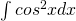This requires double angle formula:Here we introduce trigo identity:Here we have a problem!But recall we did some really similar in part 1, and notice thatis the derivative () of.

So.

Finally,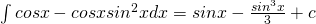Here we can apply double angle a few times to break it down before integrating.

After seeing both part 1 and part 2, you should notice some intuitive method.

Consider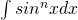and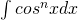.

Should n be even, we introduce the double angle formula to simplify things.
Should n be odd, we introduce the trigonometry identities and integrate. We must apply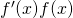method to integrate. Just saying,.

Tell me what you think in the comments section!

Not readable? Change text.